Next: Cutoffs Up: Waves in Cold Plasmas Previous: Perpendicular Wave Propagation

# Wave Propagation Through Inhomogeneous Plasmas

Up to now, we have only analyzed wave propagation through homogeneous plasmas. Let us now broaden our approach to take into account the far more realistic case of wave propagation through inhomogeneous plasmas.

Let us start off by examining a very simple case. Consider a plane electromagnetic wave, of frequency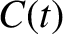, propagating along the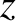-axis in an unmagnetized plasma whose refractive index,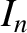, is a function of. We assume that the wave normal is initially aligned along the-axis, and, furthermore, that the wave starts off polarized in the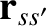-direction. It is easily demonstrated that the wave normal subsequently remains aligned along the-axis, and also that the polarization state of the wave does not change. Thus, the wave is fully described by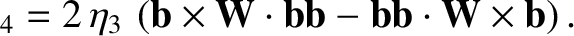(553)

and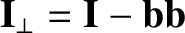(554)

It can easily be shown that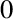and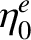satisfy the differential equations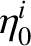(555)

and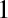(556)

respectively. Here,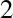is the wave-number in free space. Of course, the actual wave-number is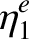.

The solution to Eq. (555) for the case of a homogeneous plasma, for whichis constant, is straightforward: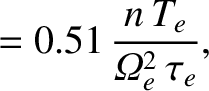(557)

whereis a constant, and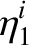(558)

The solution (557) represents a wave of constant amplitude,, and phase,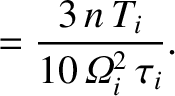. According to Eq. (558), there are, in fact, two independent waves which can propagate through the plasma. The upper sign corresponds to a wave which propagates in the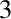-direction, whereas the lower sign corresponds to a wave which propagates in the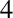-direction. Both waves propagate with the constant phase velocity.

In general, if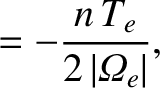then the solution of Eq. (555) does not remotely resemble the wave-like solution (557). However, in the limit in which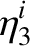is a slowly varying'' function of(exactly how slowly varying is something which will be established later on), we expect to recover wave-like solutions. Let us suppose thatis indeed a slowly varying'' function, and let us try substituting the wave solution (557) into Eq. (555). We obtain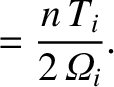(559)

This is a non-linear differential equation which, in general, is very difficult to solve. However, we note that ifis a constant then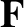. It is, therefore, reasonable to suppose that ifis a slowly varying'' function then the last term on the right-hand side of the above equation can be regarded as being small. Thus, to a first approximation Eq. (4.91) yields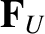(560)

and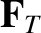(561)

It is clear from a comparison of Eqs. (559) and (561) thatcan be regarded as a slowly varying'' function ofas long as its variation length-scale is far longer than the wave-length of the wave. In other words, provided that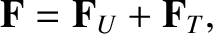.

The second approximation to the solution is obtained by substituting Eq. (561) into the right-hand side of Eq. (559):(562)

This gives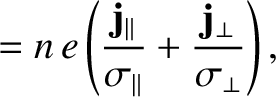(563)

where use has been made of the binomial expansion. The above expression can be integrated to give(564)

Substitution of Eq. (564) into Eq. (557) yields the final result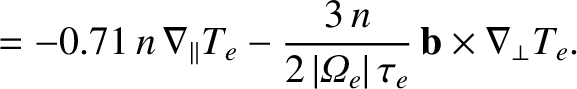(565)

It follows from Eq. (556) that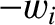(566)

Note that the second term is small compared to the first, and can usually be neglected.

Let us test to what extent the expression (565) is a good solution of Eq. (555) by substituting this expression into the left-hand side of the equation. The result is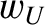(567)

This must be small compared with either term on the left-hand side of Eq. (555). Hence, the condition for Eq. (565) to be a good solution of Eq. (555) becomes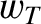(568)

The solutions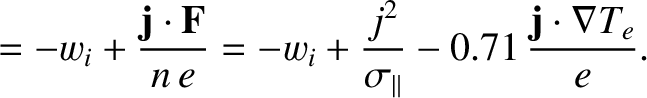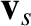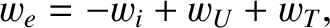(569)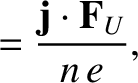(570)

to the non-uniform wave equations (555) and (556) are most commonly referred to as the WKB solutions, in honour of G. Wentzel, H.A. Kramers, and L. Brillouin, who are credited with independently discovering these solutions (in a quantum mechanical context) in 1926. Actually, H. Jeffries wrote a paper on the WKB solutions (in a wave propagation context) in 1923. Hence, some people call them the WKBJ solutions (or even the JWKB solutions). To be strictly accurate, the WKB solutions were first discussed by Liouville and Green in 1837, and again by Rayleigh in 1912. In the following, we refer to Eqs. (569)-(570) as the WKB solutions, since this is what they are most commonly known as. However, it should be understand that, in doing so, we are not making any definitive statement as to the credit due to various scientists in discovering them.

Recall, that when a propagating wave is normally incident on an interface, where the refractive index suddenly changes (for instance, when a light wave propagating through air is normally incident on a glass slab), there is generally significant reflection of the wave. However, according to the WKB solutions, (569)-(570), when a propagating wave is normally incident on a medium in which the refractive index changes slowly along the direction of propagation of the wave then the wave is not reflected at all. This is true even if the refractive index varies very substantially along the path of propagation of the wave, as long as it varies slowly. The WKB solutions imply that as the wave propagates through the medium its wave-length gradually changes. In fact, the wave-length at positionis approximately. Equations (569)-(570) also imply that the amplitude of the wave gradually changes as it propagates. In fact, the amplitude of the electric field component is inversely proportional to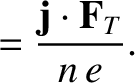, whereas the amplitude of the magnetic field component is directly proportional to. Note, however, that the energy flux in the-direction, given by the the Poynting vector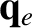, remains constant (assuming thatis predominately real).

Of course, the WKB solutions (569)-(570) are only approximations. In reality, a wave propagating into a medium in which the refractive index is a slowly varying function of position is subject to a small amount of reflection. However, it is easily demonstrated that the ratio of the reflected amplitude to the incident amplitude is of order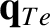. Thus, as long as the refractive index varies on a much longer length-scale than the wave-length of the radiation, the reflected wave is negligibly small. This conclusion remains valid as long as the inequality (568) is satisfied. This inequality obviously breaks down in the vicinity of a point where. We would, therefore, expect strong reflection of the incident wave from such a point. Furthermore, the WKB solutions also break down at a point where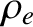, since the amplitude of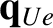becomes infinite.Next: Cutoffs Up: Waves in Cold Plasmas Previous: Perpendicular Wave Propagation
Richard Fitzpatrick 2011-03-31